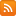CONTÁCTENOS - 91 575 78 24Estás en www.librosingenieriacivilonline.es
Si no encuentra un libro lo buscamos por Ud.
91 575 78 24

## CESTA DE LA COMPRA

### 5 TIENDAS > 1 CESTA

Tiene 0 productos en su cesta Importe total: 0
Circular Storage Tanks and Silos

# Circular Storage Tanks and Silos

## Descripción

A Design Aid for Structural Engineers Circular Storage Tanks and Silos, Third Edition effectively explains and demonstrates the concepts needed in the analysis and design of circular tanks. Tanks have to sustain high-quality serviceability over a long lifespan. This text covers computing the stresses in service in several chapters

## Características

• ISBN: 9781138073364
• Páginas: 387
• Tamaño: 17x24
• Edición:
• Idioma: Inglés
• Año: 2014

# Contenido Circular Storage Tanks and Silos

A Design Aid for Structural Engineers

Circular Storage Tanks and Silos, Third Edition effectively explains and demonstrates the concepts needed in the analysis and design of circular tanks. Tanks have to sustain high-quality serviceability over a long lifespan. This text covers computing the stresses in service in several chapters. It considers thermal stresses and the time-dependent stresses produced by creep and shrinkage of concrete and relaxation of prestressed steel. It also examines the effects of cracking and the means for its control. This text is universally applicable; no specific system of units is used in most solved examples. However, it is advantageous to use actual dimensions and forces on the structure in a small number of examples. These problems are set in SI units and Imperial units; the answers and the graphs related to these examples are given in the two systems.

What’s New in This Edition:

Presents a new chapter on recommended practice for design and construction of concrete water tanks and liquefied natural gas tanks
Includes a companion Website providing computer programs CTW and SOR
Provides material on CTW (Cylindrical Tank Walls); with simple input, it performs analysis for load combinations anticipated in the design of cylindrical walls with or without prestressing
Contains the finite-element computer program SOR (Shells of Revolution); it performs analysis for design of axisymmetrical shells of general shapes

This guide is an authoritative resource for the analysis and design of circular storage tanks and silos.

PART I Analysis

Introduction to the analysis of circular tanks

Scope

Basic assumptions

General methods of structural analysis

The displacement method

The force method

Flexibility and stiffness matrices

Moment distribution

General

Circular walls of constant thickness

Introduction

Beam-on-elastic-foundation analogy

General solution of the differential equation of the deflection of a wall of constant thickness

Characteristic parameters

Stiffness and flexibility matrices

End-rotational stiffness and carry-over factor

Fixed-end forces

Semi-infinite beam on elastic foundation and simplified equations for long cylinders

Classification of beams on elastic foundation and circular walls as long or short

Examples

General

Circular walls of varying thickness

Introduction

Finite-difference equations

Boundary conditions and reactions

Generation of simultaneous equations

Sudden change in thickness

Examples

Flexibility and stiffness of circular walls of variable thickness

Effect of temperature

General

Design tables and examples of their use

Introduction

Description of design tables

Variables

Arbitrary edge conditions

Examples

Long cylinders

Poisson’s ratio

Beams on elastic foundations

General

Finite-element analysis

Introduction

Nodal displacements and nodal forces

Transformation of stiffness matrix

Displacement interpolations

Stress resultants

Stiffness matrix of individual element

Analysis for effect of temperature

Assemblage of stiffness matrices and load vectors

Nodal forces

Examples

Time-dependent effects

Introduction

Creep and shrinkage of concrete

Relaxation of prestressed steel

Basic equations for stress and strain distributions in a homogeneous section

Time-dependent stress and strain in a section

Normal force N and bending moment M due to prestressing

Time-dependent internal forces

Thermal stresses

Introduction

Effects of temperature variation in a cylindrical wall

Linear temperature variation through wall thickness

Thermal internal forces in deep tanks and silos

Internal forces due to shrinkage of concrete

Significance of linear analysis

Optimum design of prestressing

Introduction

Trapezoidal distribution of circumferential prestressing

Design objectives

Design of circumferential prestress distribution

Effects of cracking of concrete

Introduction

Stress and strain in a cracked section

Decompression forces

Equilibrium equations

Rectangular section analysis

Tension stiffening

Crack width

Time-dependent strain and stress in cracked sections

Influence of cracking on internal forces

Control of cracking in concrete tanks and silos

Introduction

Causes of cracking

Tensile strength of concrete

Force-induced cracking

Displacement-induced cracking

Cracking by bending: force-induced and displacement-induced

Motives for crack control

Means of controlling cracking

Minimum reinforcement to avoid yielding of reinforcement

Amount of reinforcement to limit crack width: displacement-induced cracking

Change in steel stress at cracking

Part II Design tables

Tables for analysis of circular walls of thickness varying linearly from top to bottom

Appendices:

Stiffness and fixed-end forces for circular an annular plates

Computer program CTW (cylindrical tank walls)

Computer program SOR (shells of revolution)

Index

## ACERCA DE LA EMPRESA